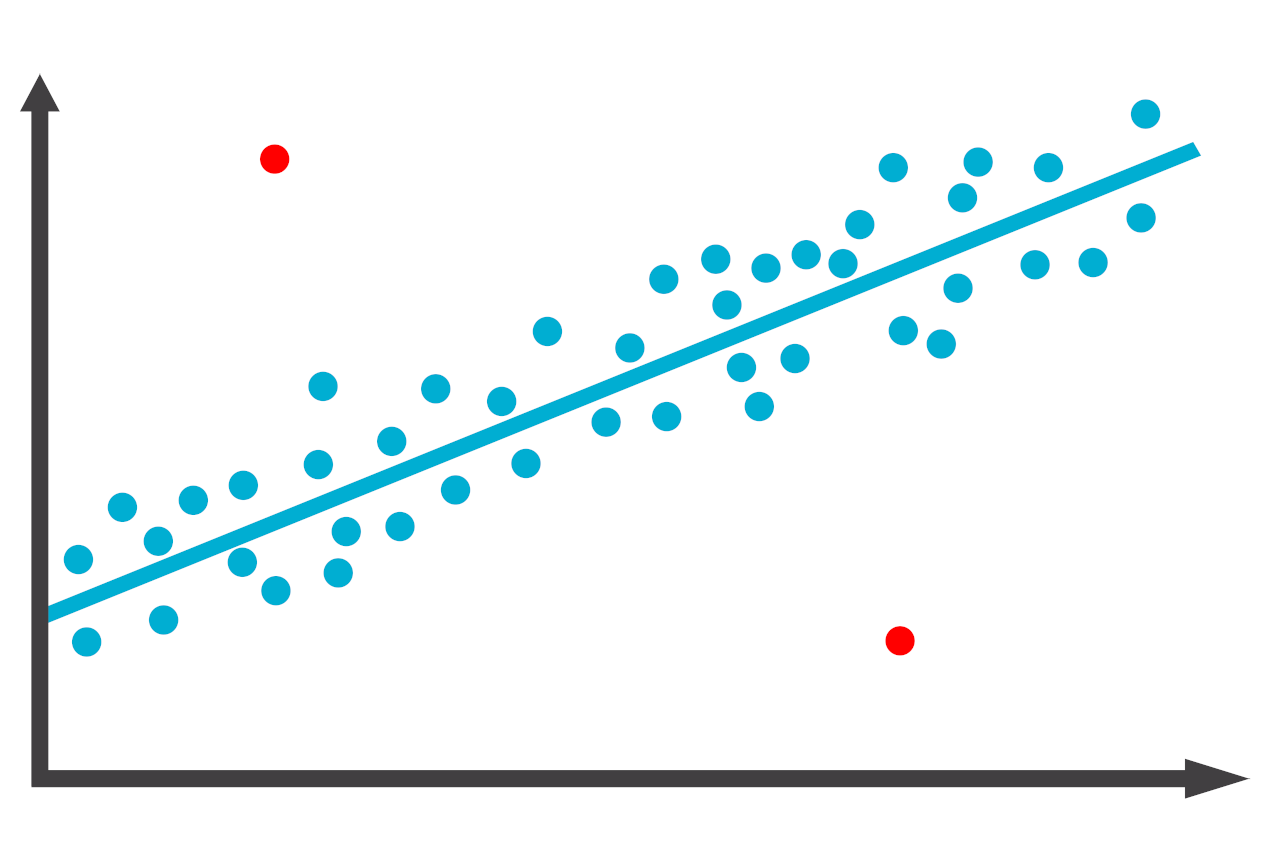# Outlier Calculator

Find the outliers in a data set by entering the numbers in the calculator below.

Separate numbers using a comma (,)

## Outliers:

### Steps to Solve

#### Step One: Find the Quartiles

The first step is to find the quartiles for the data set.

For this data set, the quartiles are:

Q1 = ?
Q3 = ?

#### Step Two: Find the Interquartile Range

The interquartile range is the difference between the first and third quartiles.

IQR = Q3 - Q1

#### Step Three: Find the Fences

The next step is to set up a fence beyond the first and third quartiles using the interquartile range.

Lower Fence = Q1 - (1.5 × IQR)

Upper Fence = Q3 + (1.5 × IQR)

#### Step Four: Find the Outliers

Any numbers in the data that are above or below the fences are outliers.

## How to Find Outliers

In statistics, outliers are observations that lie an abnormal distance from other values in a set of data. These data points are considered unusual and are often problematic in statistical analyses because they tend to distort the results.

There are a few methods that you can use to find outliers in the data.

### Method One: Visually Identify Outliers

The first method is to visually identify outliers. Looking at the scatter plot graph below, it’s easy to spot the outliers shown in red.Looking at the graph, two values look unusually compared to the other data, which follow the line closely and are outliers in the data.

### Method Two: Using the IQR Method

The second method to find outliers in the data is to use the interquartile range method. To use this method, find the quartiles and interquartile range for the data.

Then, using the quartiles and interquartile range, set fences beyond the quartiles. Any values outside of these fences are considered outliers.

You can find the fences using the following formula:

Lower Fence = Q1 – (1.5 × IQR)
Upper Fence = Q3 + (1.5 × IQR)

Any values in the data that are outside of the fences are outliers.

### Method Three: Using Z-Scores

The third method to find outliers in the data is to use z-scores. To use this method, calculate z-scores for each observation.

The further the value’s z-score is from zero, the more unusual the value is. Values with a z-score above 3.0 or below -3.0 are considered outliers using this method.